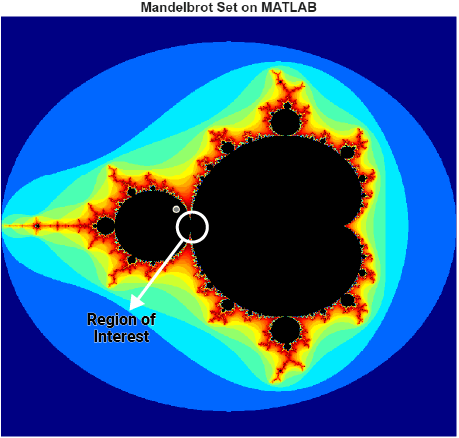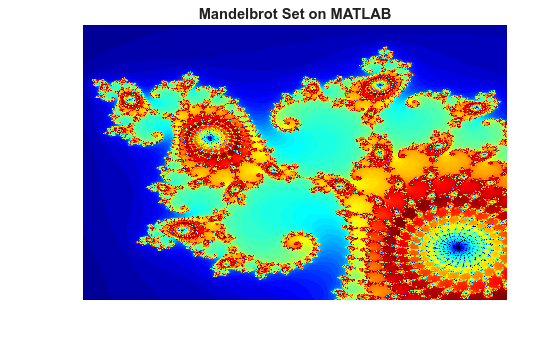# GPU Code Generation: The Mandelbrot Set

This example shows how to generate CUDA® code from a simple MATLAB® function by using GPU Coder™. A Mandelbrot set implementation by using standard MATLAB commands acts as the entry-point function. This example uses the codegen command to generate a MEX function that runs on the GPU. You can run the MEX function to check for run-time errors.

### Third-Party Prerequisites

Required

This example generates CUDA MEX and has the following third-party requirements.

• CUDA enabled NVIDIA® GPU and compatible driver.

Optional

For non-MEX builds such as static, dynamic libraries or executables, this example has the following additional requirements.

### Verify GPU Environment

To verify that the compilers and libraries necessary for running this example are set up correctly, use the coder.checkGpuInstall function.

envCfg = coder.gpuEnvConfig('host'); envCfg.BasicCodegen = 1; envCfg.Quiet = 1; coder.checkGpuInstall(envCfg); 

### Mandelbrot Set

The Mandelbrot set is the region in the complex plane consisting of the valuesfor which the trajectories defined byremain bounded at. The overall geometry of the Mandelbrot set is shown in the figure. This view does not have the resolution to show the richly detailed structure of the fringe just outside the boundary of the set.### Define Input Regions

Pick a set of limits that specify a highly zoomed part of the Mandelbrot set in the valley between the main cardioid and thebulb to its left. A 1000x1000 grid ofandis created between these two limits. The Mandelbrot algorithm is then iterated at each grid location. An iteration number of 500 is enough to render the image in full resolution.

maxIterations = 500; gridSize = 1000; xlim = [-0.748766713922161, -0.748766707771757]; ylim = [ 0.123640844894862, 0.123640851045266]; x = linspace( xlim(1), xlim(2), gridSize ); y = linspace( ylim(1), ylim(2), gridSize ); [xGrid,yGrid] = meshgrid( x, y ); 

### The Mandelbrot Entry-Point Function

The mandelbrot_count.m entry-point function contains a vectorized implementation of the Mandelbrot set based on the code provided in the e-book Experiments with MATLAB by Cleve Moler. The %#codegen directive turns on MATLAB for code generation error checking. When GPU Coder encounters the coder.gpu.kernelfun pragma, it attempts to parallelize all the computation within this function, and then maps it to the GPU.

type mandelbrot_count 
function count = mandelbrot_count(maxIterations, xGrid, yGrid) %#codegen % Copyright 2016-2019 The MathWorks, Inc. z0 = xGrid + 1i*yGrid; count = ones(size(z0)); % Map computation to GPU. coder.gpu.kernelfun; z = z0; for n = 0:maxIterations z = z.*z + z0; inside = abs(z)<=2; count = count + inside; end count = log(count); 

### Test the Functionality of mandelbrot_count

Run the mandelbrot_count function with the xGrid, yGrid values that were previously generated, and then plot the results.

count = mandelbrot_count(maxIterations, xGrid, yGrid); figure(2), imagesc( x, y, count ); colormap( [jet();flipud( jet() );0 0 0] ); title('Mandelbrot Set on MATLAB'); axis off### Generate CUDA MEX for the Function

To generate CUDA MEX for the mandelbrot_count function, create a GPU code configuration object and run the codegen command. Because of architectural differences between the CPU and GPU, numeric verification does not always match. This scenario is true when using the single data type in your MATLAB code and performing accumulation operations on these single data type values. Like this Mandelbrot example even the double data types cause numeric errors. One reason for this mismatch is that the GPU floating-point units use fused Floating-point Multiply-Add (FMAD) instructions and the CPU does not use these instructions. The fmad=false option that is passed to the nvcc compiler turns off this FMAD optimization.

cfg = coder.gpuConfig('mex'); cfg.GpuConfig.CompilerFlags = '--fmad=false'; codegen -config cfg -args {maxIterations,xGrid,yGrid} mandelbrot_count 
Code generation successful: To view the report, open('codegen/mex/mandelbrot_count/html/report.mldatx'). 

### Run the MEX Function

After generating a MEX function, verify that it has the same functionality as the original MATLAB entry-point function. Run the generated mandelbrot_count_mex and plot the results.

countGPU = mandelbrot_count_mex(maxIterations, xGrid, yGrid); figure(2), imagesc( x, y, countGPU ); colormap( [jet();flipud( jet() );0 0 0] ); title('Mandelbrot Set on GPU'); axis off